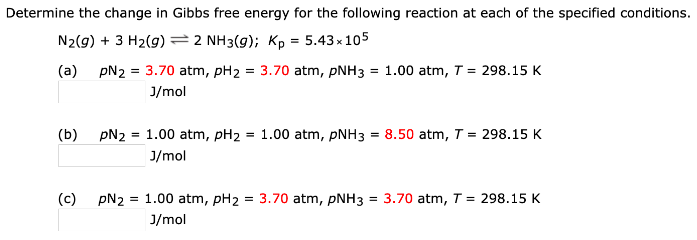# Determine the change in Gibbs free energy for the following reaction at each of the specified conditions. N2(g) + 3 H2(g) <-> 2 NH3(g); Kp = 5.43 x 10^5 (a) pN2 = 3.70 atm, pH2 = 3.70 atm, pNH3 = 1.00 atm, T = 298.15 K (b) pN2 = 1.00 atm, pH2 = 1.00 atm, pNH3 = 8.50 atm, T = 298.15 K (c) pN2 = 1.00 atm, pH2 = 3.70 atm, pNH3 = 3.70 atm, T = 298.15 K